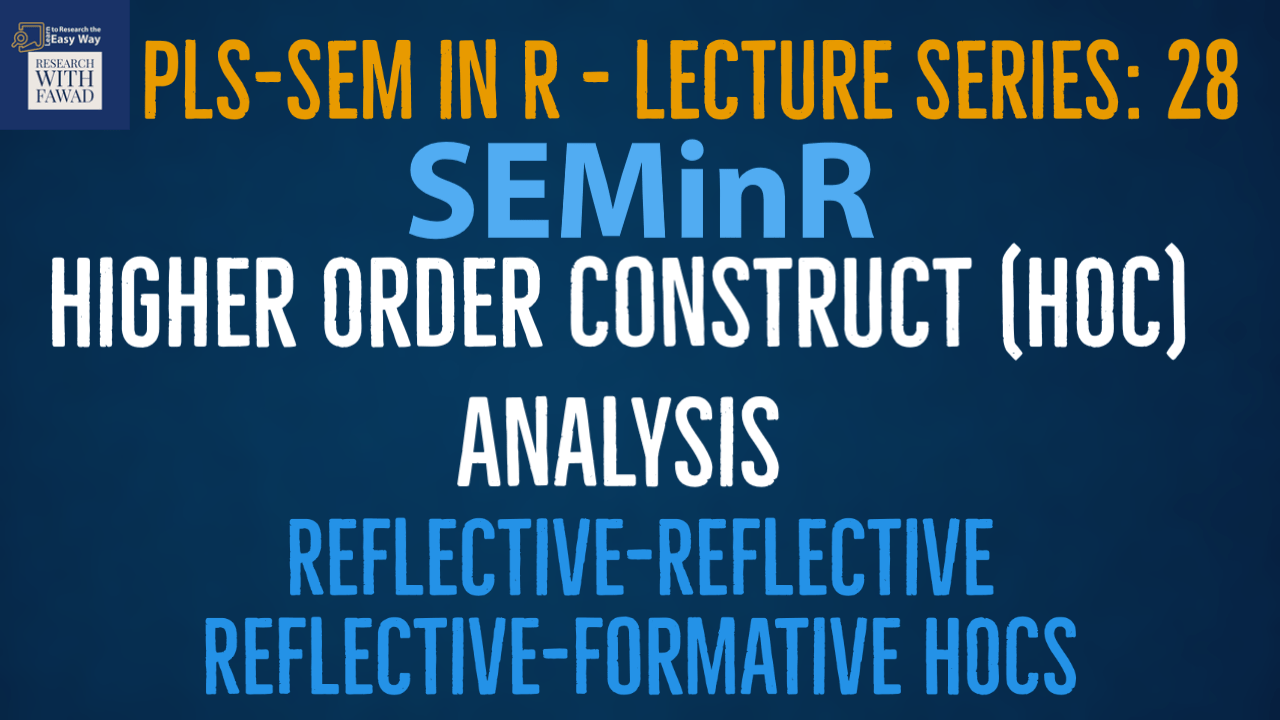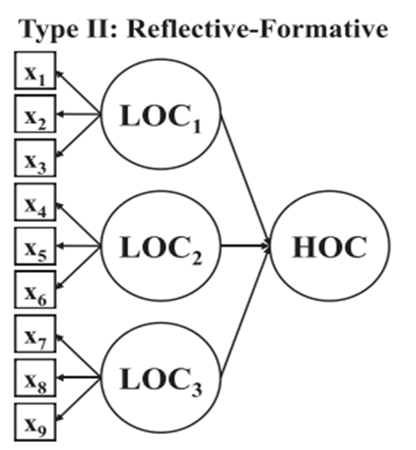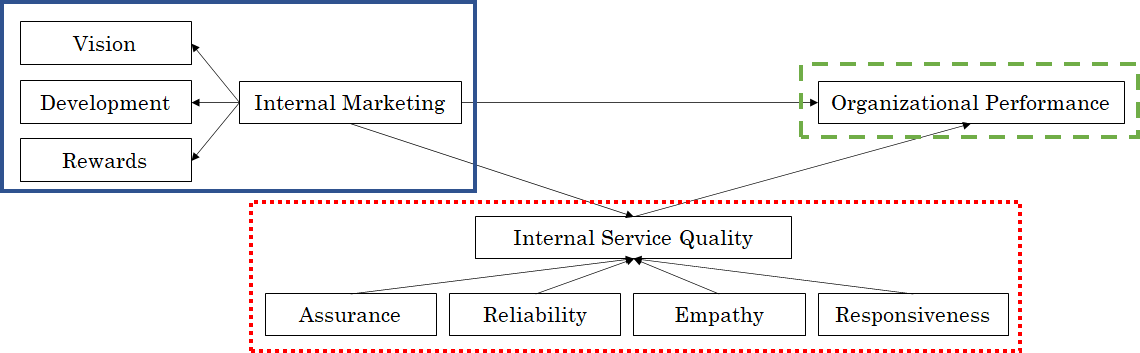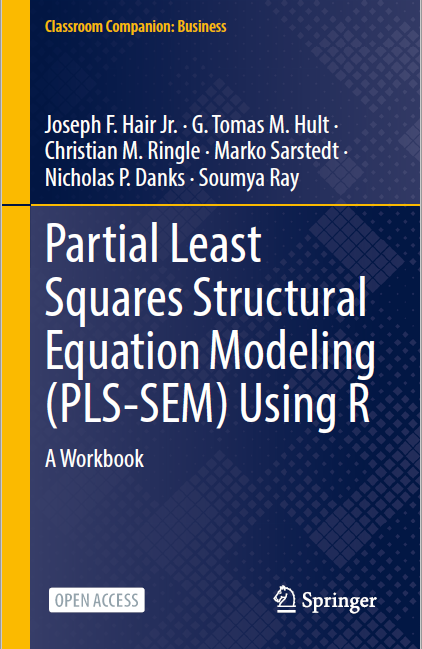# SEMinR Lecture Series - REF-REF and REF-FOR Higher Order Construct Analysis### SEMinR Lecture Series

This session is focused on how to estimate and validate REF-REF and REF-FOR Higher Order Constructs using SEMinR in Cran R

## Reflective-Formative Higher Order Construct

• A Reflective-Formative model is one where the first order has reflective indicators while in the second order the dimensions are formative. Here is how it looks## Steps in Analyzing Reflective-Reflective and Reflective-Formative Higher Order Construct

Step 1: Asses the Reliability and Validity for all the Lower Order Reflective Constructs (No matter whether they belong to a Reflective or Formative Higher Order Construct)

Step 2: Assess the Reliability and Validity of the Higher Order Reflective-Reflective Construct (At the Second Order/Level). Also include any reflective LOCs when assessing the Reliability and Validity of the Higher Order Ref-Ref model.

Step 3: Assess the Validity of the Higher/Second Order Formative Construct (Assess Collinearity, Indicators Weight, Factor Loadings).

Step 4: Evaluate the Structural Model

• Collinearity Diagnostics
• Assess the Structural Relationships
• Explanatory Power

## Example

• The example model for this session is• Blue Solid Line: Reflective-Reflective
• Red Dots: Reflective-Formative
• Green Dashes: Lower Order Construct

## Step 1: SEMinR Code

``````#One Reflective and One Formative - No Error
library(seminr)
# Create measurement model
simple_mm = constructs(
composite("Vision", multi_items("VIS", 1:4)),
composite("Development", multi_items("DEV", 1:7)),
composite("Rewards", multi_items("RW", 1:4)),
composite("Assurance", multi_items("ASR", 1:4)),
composite("Reliability", multi_items("REL", 1:5)),
composite("Empathy", multi_items("EMP", 1:5)),
composite("Responsiveness", multi_items("RES", 1:4)),
composite("Organizational Performance", multi_items("OP", 1:5)))
# Create structural model
simple_sm = relationships(
paths(from = c("Vision", "Development", "Rewards"), to = "Organizational Performance"),
paths(from = c("Vision", "Development", "Rewards"), to = c("Assurance", "Reliability", "Empathy", "Responsiveness")),
paths(from = c("Assurance", "Reliability", "Empathy", "Responsiveness"), to = "Organizational Performance"))
# Estimate the model
simple_model=estimate_pls(data=datas, measurement_model=simple_mm, structural_model=simple_sm)
plot(simple_model)
# Summarize the model results
summary_simple = summary(simple_model)
#Reliability
summary_simple\$reliability
#F&L Criteria
summary_simple\$validity\$fl_criteria
#HTMT
summary_simple\$validity\$htmt
#Collinearity analysis
summary_simple\$validity\$vif_items``````

## Step 2: SEMinR Code

``````#One Reflective and One Formative - No Error
library(seminr)
# Create measurement model
simple_mm = constructs(
composite("Vision", multi_items("VIS", 1:4)),
composite("Development", multi_items("DEV", 1:7)),
composite("Rewards", multi_items("RW", 1:4)),
higher_composite("IM", c("Vision","Development","Rewards"), method = "two stage"),
composite("Assurance", multi_items("ASR", 1:4)),
composite("Reliability", multi_items("REL", 1:5)),
composite("Empathy", multi_items("EMP", 1:5)),
composite("Responsiveness", multi_items("RES", 1:4)),
higher_composite("ISQ", c("Assurance","Reliability", "Empathy", "Responsiveness"), method = "two stage", weights = mode_B),
composite("Organizational Performance", multi_items("OP", 1:5)))
# Create structural model
simple_sm = relationships(
paths(from = "IM", to = "ISQ"),
paths(from = "ISQ", to = "Organizational Performance"),
paths(from = "IM", to = "Organizational Performance"))
# Estimate the model
simple_model=estimate_pls(data=datas, measurement_model=simple_mm, structural_model=simple_sm)
plot(simple_model)
# Summarize the model results
summary_simple = summary(simple_model)
#Reliability
summary_simple\$reliability
#F&L Criteria
summary_simple\$validity\$fl_criteria
#HTMT
summary_simple\$validity\$htmt
#Collinearity analysis
summary_simple\$validity\$vif_items

# Bootstrap the model on the PLS Estimated Model
boot_model <- bootstrap_model(
seminr_model = simple_model,
nboot = 1000,
cores = parallel::detectCores(),
seed = 123)
# Store the summary of the bootstrapped model
# alpha sets the specified level for significance, i.e. 0.05
summary_boot <- summary(boot_model, alpha = 0.10)
summary_boot\$bootstrapped_weights
summary_simple\$validity\$vif_items
specific_effect_significance(boot_seminr_model = boot_model, from = "IM", through = "ISQ", to = "Organizational Performance", alpha = 0.05)
summary_boot\$bootstrapped_paths
plot(boot_model)``````

## Reference

Hair Jr, J. F., Hult, G. T. M., Ringle, C. M., Sarstedt, M., Danks, N. P., & Ray, S. (2021). Partial Least Squares Structural Equation Modeling (PLS-SEM) Using R: A Workbook.The tutorials on SEMinR are based on the mentioned book. The book is open source and available for download under this link.